# Types of Angles

In this article, you will learn about various types of Angles: Acute angle, Obtuse angle, Right angle, Straight angle, reflex angle, complete angle, complementary angle and more.

## What is Angle?

An angle is formed by two rays with a common endpoint. We measure the size of an angle in DEGREES. Sides and Vertex are the two components of an angle.

The two rays forming the angle are called sides. The common endpoint of the rays is called the vertex.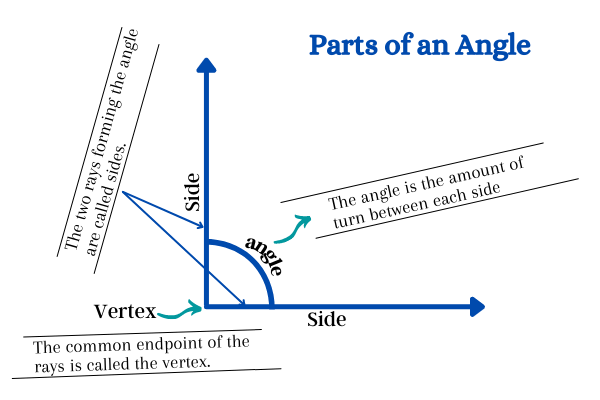## Types of Angles

There are mainly SIX types of angles based on their measure of the angle. They include:

1. Acute Angles
2. Right Angles
3. Obtuse Angles
4. Straight Angles
5. Reflex Angles
6. Complete Angles

### 1. Acute AngleAn acute angle is an angle that lies between 0° and 90°. It means an acute angle is greater than 0° but less than 90°. In the above picture, the angle ∠PQR formed by the intersection of PQ and QR which measures 45°. Thus ∠PQR=45° is an acute angle.  Common examples of acute angles include 15°, 30°, 45°, 80°, etc.

### 2. Right Angle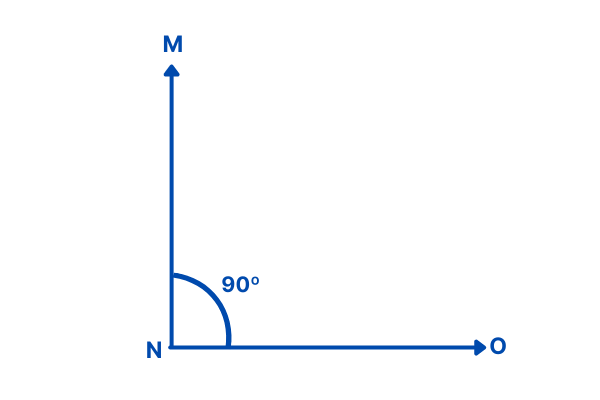A right angle is an angle whose measure is exactly 90°. It is mainly formed when two straight lines are perpendicular to each other. ∠MNO = 90°.

### 3. Obtuse Angle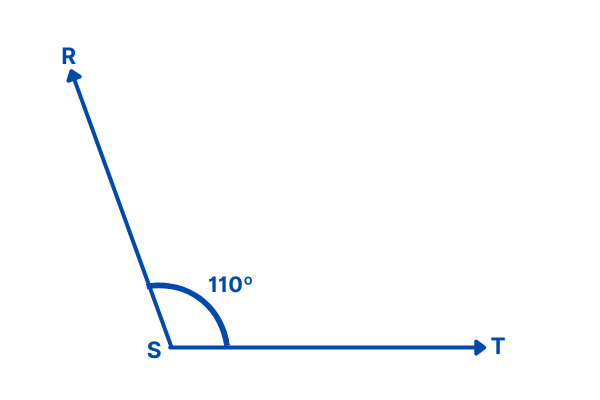An obtuse angle is an angle that lies between 90° and 180°. It means an obtuse angle is greater than 90° but less than 180°. In the above picture, the angle ∠RST formed by the intersection of RS and ST which measures 110°. Thus ∠RST=110° is an obtuse angle.  Common examples of obtuse angles include 100°, 115°, 145°, 160°, etc.

### 4. Straight Angle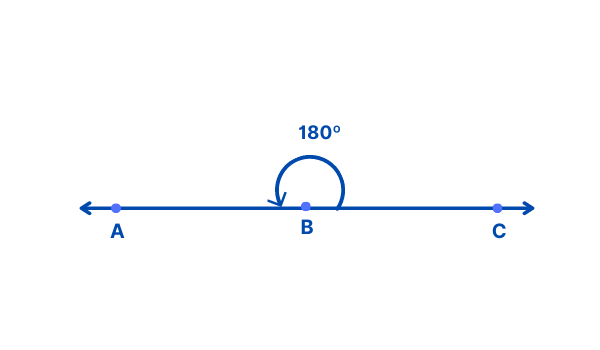A straight angle is an angle whose measure is exactly 180°. It is one-half of a whole circle. Straight angle is a combination of acute angle and obtuse angle.

Acute angle = Straight angle – Obtuse angle
Example: 180° – 110° = 70° ( acute angle )

Obtuse angle = Straight angle – Acute angle
Example: 180° – 40° = 140° ( obtuse angle )

### 5. Reflex AngleA reflex angle is an angle that lies between 180° and 360º. It means a reflex angle is greater than 180° but less than 360°.  [Between straight angle (180º) and a whole turn (360º)]. In the above picture, the angle ∠RST formed by the intersection of RS and ST which measures 220°. Thus ∠RST=220° is an reflex angle. Common examples of obtuse angles include: 190°, 215°, 345°, 350°, etc.

### 6. Complete Angle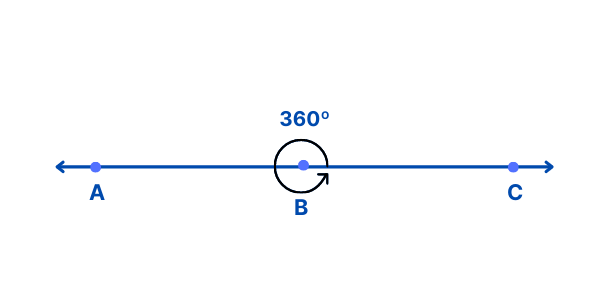A complete angle is an angle whose measure is exactly 360°. It is one complete revolution equal to 360°. It is also called full rotation or full angle.

## Other Types of angles: How to name a pairs of angles

Apart from the types of angles mentioned above, different types of angles are known as pair angles. They include:

### Complementary AngleIf the sum of two angles is equal to 90°, then they are called complementary angles. One of the complementary angles is said to be the complement of the other.

In the above picture, ∠PQR + ∠RQS = 55° + 35° = 90°.Example: 53°
To determine the complement, subtract the given angle from 90°.

90° – 53° = 37°,
Therefore, the complement of 53° is 37°.

### Supplementary AngleIf the sum of two angles is equal to 180°, then they are supplementary angles. One of the supplementary angles is said to be the supplement of the other.
In the above picture, ∠ABC + ∠CBD = 60° + 120° = 180°.

Example: 75°
To determine the supplement, subtract the given angle from 180°.

180° – 75° = 105°,
Therefore, the supplement of 75° is 105°.

### Linear Pair AnglesA pair of adjacent angles whose sum is a straight angle, i.e., 180°, is called a linear pair. It means The two angles of a linear pair are always supplementary.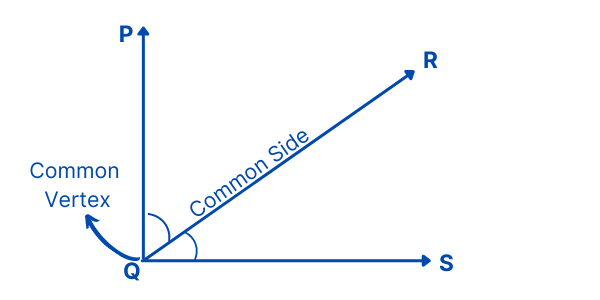Two angles that share a common side and a common vertex (corner point) are called adjacent angles.

### Vertically Opposite Angles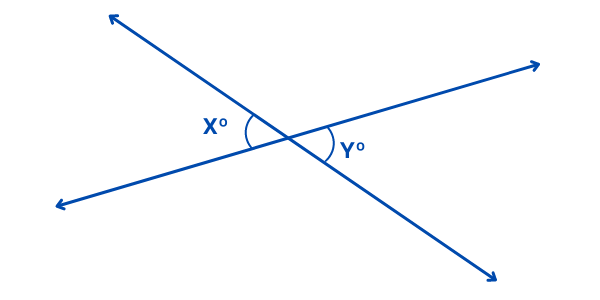Vertical opposite angles are formed when two lines intersect each other. It is called vertically opposite angles because due to intersection, the angles are opposite to each other.

Translate »
Scroll to Top